/

### The Gaussian copula

The Gaussian copula is the copula that underlies the multivariate normal distribution.

 Copula name Gaussian copula Common notation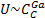Parameters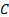, a non-negative definite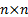matrix, i.e. a matrix that can correspond to a correlation matrix Domain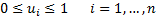Copula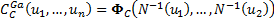where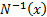is the inverse normal function and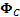is the cumulative distribution function of the multivariate normal distribution defined by a covariance matrix equal toKendall’s rank correlation coefficient (for bivariate case),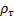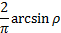Where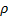is the correlation coefficient between the two variables Coefficient of upper tail dependence,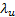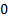(unless the correlation matrix exhibits perfect positive or negative dependence) Coefficient of lower tail dependence,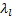(unless the correlation matrix exhibits perfect positive or negative dependence) Other comments The Spearman rank correlation coefficient is given by: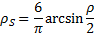whereis the (normal) correlation coefficient between the two variables.   If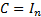(theidentity matrix) then we obtain the independence copula.

Nematrian web functions

Functions relating to the above distribution in the two dimensional case may be accessed via the Nematrian web function library by using a DistributionName of “Gaussian Copula (2d)”. For details of other supported probability distributions see here.